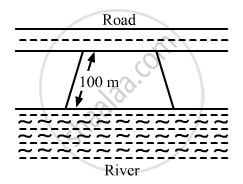Share

# Mohan Wants to Buy a Trapezium Shaped Field. Its Side Along the River is Parallel and Twice the Side Along the Road. - Mathematics

Course
ConceptArea of Trapezium

#### Question

Mohan wants to buy a trapezium shaped field. Its side along the river is parallel and twice the side along the road. If the area of this field is 10500 m2 and the perpendicular distance between the two parallel sides is 100 m, find the length of the side along the river.#### SolutionGiven:

Area of the trapezium shaped field = 10500 m2

It is also given that the length of the side along the river is double the length of the side along the road.

Let us suppose the length of the side along the road to be x.

Then, the length of the side along the river =$2\times x = 2x$

And, the perpendicular distance between these parallel sides =100 m

Area of trapezium $=\frac{1}{2}\times(\text{ Sum of the parallel sides })\times(\text{ Perpendicular distance })$

$10500 = \frac{1}{2}\times(2x+x)\times(100)$

$10500=50\times(3x)$

$3x=\frac{10500}{50}=210$

$x=\frac{210}{3}=70 m$

$\therefore\text{ Length of the side along the river }= 2\times x= 2\times70=140 m$

Is there an error in this question or solution?

#### APPEARS IN

RD Sharma Solution for Mathematics for Class 8 by R D Sharma (2019-2020 Session) (2017 to Current)
Chapter 20: Mensuration - I (Area of a Trapezium and a Polygon)
Ex. 20.2 | Q: 14 | Page no. 23

#### Video TutorialsVIEW ALL 

Solution Mohan Wants to Buy a Trapezium Shaped Field. Its Side Along the River is Parallel and Twice the Side Along the Road. Concept: Area of Trapezium.
S